/ #Machine Learning #Propensity Modelling

# Propensity Modelling - Using h2o and DALEX to Estimate the Likelihood of Purchasing a Financial Product - Estimate Several Models and Compare Their Performance Using a Model-agnostic Methodology

In this day and age, a business that leverages data to understand the drivers of its customers’ behaviour has a true competitive advantage. Organisations can dramatically improve their performance in the market by analysing customer level data in an effective way and focus their efforts towards those that are more likely to engage.

One trialled and tested approach to tease out this type of insight is Propensity Modelling, which combines information such as a customers’ demographics (age, race, religion, gender, family size, ethnicity, income, education level), psycho-graphic (social class, lifestyle and personality characteristics), engagement (emails opened, emails clicked, searches on mobile app, webpage dwell time, etc.), user experience (customer service phone and email wait times, number of refunds, average shipping times), and user behaviour (purchase value on different time-scales, number of days since most recent purchase, time between offer and conversion, etc.) to estimate the likelihood of a certain customer profile to performing a certain type of behaviour (e.g. the purchase of a product).

Once you understand the probability of a certain customer to interact with your brand, buy a product or sign up for a service, you can use this information to create scenarios, be it minimising marketing expenditure, maximising acquisition targets, and optimise email send frequency or depth of discount.

## Project Structure

In this project I’m analysing the results of a bank direct marketing campaign to sell term deposits in order to identify what type of customer is more likely to respond. The marketing campaigns were based on phone calls and more than one contact to the same client was required at times.

First, I am going to carry out an extensive data exploration and use the results and insights to prepare the data for analysis.

Then, I’m estimating a number of models and assess their performance and fit to the data using a model-agnostic methodology that enables to compare traditional “glass-box” models and “black-box” models.

Last, I’ll fit one final model that combines findings from the exploratory analysis and insight from models’ selection and use it to run a revenue optimisation.

## Modelling strategy

``````library(tidyverse)
library(skimr)
library(h2o)
library(DALEX)
library(knitr)
library(tictoc)
``````

In order to stick to a reasonable project running time, I’ve opted for h2o as my modelling platform as it offers a number of advantages:

• it’s very easy to use and you can estimate several Machine Learning models in no time

• it does not require to pre-treat character/factor variables by “binarising” them (this is done “internally”), which further reduces data formatting time

• it has a functionality that takes care of the class imbalance highlighted in the Data Exploration phase - I simply set `balance_classes` = TRUE in the model specification, more on this later on

• cross-validation can be enabled without the need for a separate `validation frame` to be “carved out” of the training set

• hyper-parameters fine tuning (a.k.a. grid search) can be implemented alogside a number of strategies that ensure running time is capped without compromising on performance

### Creating the training and validation sets

Before I begin, I load the cleansed data saved at the end of the exploratory analysis

``````# Loading clensed data
``````

I’m starting by creating a randomised training and validation set with `rsample`

``````set.seed(seed = 1975)

train_test_split <-
rsample::initial_split(
data = data_final,
prop = 0.80
)

train_test_split

## <32951/8237/41188>
``````

Of the 41,188 total customers, 32,951 have been assigned to the training set and 8,237 to the test set. I save them as `train_tbl` and `test_tbl`.

``````train_tbl <- train_test_split %>% rsample::training()
test_tbl  <- train_test_split %>% rsample::testing()
``````

### Building models with h2o

The next step is to start a h2o cluster. I specify the size of the memory cluster to “16G” to help speed things up a bit and turn off the progress bar.

``````# initialize h2o session and switch off progress bar
h2o.no_progress()
h2o.init(max_mem_size = "16G")

##
## H2O is not running yet, starting it now...
##
## Note:  In case of errors look at the following log files:
##     C:\Users\LENOVO\AppData\Local\Temp\RtmpoJnEwn/h2o_LENOVO_started_from_r.out
##     C:\Users\LENOVO\AppData\Local\Temp\RtmpoJnEwn/h2o_LENOVO_started_from_r.err
##
##
## Starting H2O JVM and connecting: ... Connection successful!
##
## R is connected to the H2O cluster:
##     H2O cluster uptime:         7 seconds 431 milliseconds
##     H2O cluster timezone:       Europe/Berlin
##     H2O data parsing timezone:  UTC
##     H2O cluster version:        3.28.0.4
##     H2O cluster version age:    2 months and 4 days
##     H2O cluster name:           H2O_started_from_R_LENOVO_frm928
##     H2O cluster total nodes:    1
##     H2O cluster total memory:   14.22 GB
##     H2O cluster total cores:    4
##     H2O cluster allowed cores:  4
##     H2O cluster healthy:        TRUE
##     H2O Connection ip:          localhost
##     H2O Connection port:        54321
##     H2O Connection proxy:       NA
##     H2O Internal Security:      FALSE
##     H2O API Extensions:         Amazon S3, Algos, AutoML, Core V3, TargetEncoder, Core V4
##     R Version:                  R version 3.6.3 (2020-02-29)
``````

Next, I sort out response and predictor variables sets. For a classification to be performed, I need to ensure that the response variable is a factor (otherwise h2o will carry out a regression). This was sorted out during the data clensing and formatting phase.

``````# response variable
y <- "subscribed"

# predictors set: remove response variable
x <- setdiff(names(train_tbl %>% as.h2o()), y)
``````

### Fitting the models

For this project I’m estimating a Generalised Linear Model (a.k.a. Elastic Net), a Random Forest (which h2o refers to at Distributed Random Forest) and a Gradient Boosting Machine (or GBM).

To implement a grid search for the `tree-based` models (DRF and GBM), I need to set up a random grid to search for optimal hyper-parameters for the `h2o.grid()` function . To do so, I define the search parameters to be passed to the `hyper_params`argument:

• `sample_rate` is used to set the row sampling rate for each tree

• `col_sample_rate_per_tree` defines the column sampling for each tree

• `max_depth` specifies the maximum tree depth

• `min_rows` to fix the minimum number of observations per leaf

• `mtries`(DRF only) indicates the columns to randomly select on each node of the tree

• `learn_rate`(GBM only) specifies the rate at which the model learns when building a model

``````# DRF hyperparameters
hyper_params_drf <-
list(
mtries                   = seq(2, 5, by = 1),
sample_rate              = c(0.65, 0.8, 0.95),
col_sample_rate_per_tree = c(0.5, 0.9, 1.0),
max_depth                = seq(1, 30, by = 3),
min_rows                 = c(1, 2, 5, 10)
)

# GBM hyperparameters
hyper_params_gbm <-
list(
learn_rate               = c(0.01, 0.1),
sample_rate              = c(0.65, 0.8, 0.95),
col_sample_rate_per_tree = c(0.5, 0.9, 1.0),
max_depth                = seq(1, 30, by = 3),
min_rows                 = c(1, 2, 5, 10)
)
``````

I also set up a second list for the `search_criteria` argument, which helps to manage the models’ estimation running time:

• The `strategy` argument is set to RandomDiscrete for the search to randomly select a combination from the grid search parameters

• Setting `stopping_metric` to AUC as the error metric for early stopping - the models will stop building new trees when the metric ceases to improve

• With `stopping_rounds` I’m specifying the number of training rounds before early stopping is considered

• I’m using `stopping_tolerance` to set minimal improvement needed for the training process to continue

• `max_runtime_secs` restricts the search time to one hour per model

``````search_criteria_all <-
list(
strategy           = "RandomDiscrete",
stopping_metric    = "AUC",
stopping_rounds    = 10,
stopping_tolerance = 0.0001,
max_runtime_secs   = 60 * 60
)
``````

At last, I can set up the models’ formulations. Note that all models have 2 parameters in common:

• the `nfolds` parameter, which enables cross-validation to be carried out without the need for a validation_frame - if set to 5 for instance, it will perform a 5-fold cross-validation

• the `balance_classes` parameter is set to TRUE to account for the imbalance in the target variable highlighted during the exploratory analysis. When enabled, h2o will either under-sample the majority class or oversample the minority class.

``````# elastic net model
glm_model <-
h2o.glm(
x               = x,
y               = y,
training_frame  = train_tbl %>% as.h2o(),
balance_classes = TRUE,
nfolds          = 10,
family          = "binomial",
seed            = 1975
)

# random forest model
drf_model_grid <-
h2o.grid(
algorithm       = "randomForest",
x               = x,
y               = y,
training_frame  = train_tbl %>% as.h2o(),
balance_classes = TRUE,
nfolds          = 10,
ntrees          = 1000,
grid_id         = "drf_grid",
hyper_params    = hyper_params_drf,
search_criteria = search_criteria_all,
seed            = 1975
)

gbm_model_grid <-
h2o.grid(
algorithm       = "gbm",
x               = x,
y               = y,
training_frame  = train_tbl %>% as.h2o(),
balance_classes = TRUE,
nfolds          = 10,
ntrees          = 1000,
grid_id         = "gbm_grid",
hyper_params    = hyper_params_gbm,
search_criteria = search_criteria_all,
seed            = 1975
)
``````

I sort the tree-based model by AUC score and retrieve the lead models from the grid

``````# Get the DRM grid results, sorted by AUC
drf_grid_perf <-
h2o.getGrid(grid_id     = "drf_grid",
sort_by    = "AUC",
decreasing = TRUE)

# Fetch the top DRF model, chosen by validation AUC
drf_model <-
h2o.getModel(drf_grid_perf@model_ids[])

# Get the GBM grid results, sorted by AUC
gbm_grid_perf <-
h2o.getGrid(grid_id     = "gbm_grid",
sort_by    = "AUC",
decreasing = TRUE)

# Fetch the top GBM model, chosen by validation AUC
gbm_model <-
h2o.getModel(gbm_grid_perf@model_ids[])
``````

ALWAYS REMEMBER that h2o requires a live connection to their servers to operate properly. For that reason it is important that you save away all models you estimate in your current live connection so that they can be accessed at any time.

``````# set path to get around model path being different from project path
path = "../03_models/"

# Save GLM model
h2o.saveModel(glm_model, path)

# Save RF model
h2o.saveModel(drf_model, path)

# Save GBM model
h2o.saveModel(gbm_model, path)
``````

This is especially important in the event of momentary loss of web connection, which I figured out to my expense. In case connection is lost and you have to restart the h2o cluster, models that are still in the RStudio environment but estimated in the previous session are NOT recognised as part of the current cluster and need to be re-estimated. This could be a little annoying, especially when you fit complex models that require extensive running time to converge.

## Performance assessment

There are many libraries (like IML, PDP, VIP, and DALEX to name but the more popular) that help with Machine Learning Interpretability, feature explanation and general performance assessment and they all have gained in popularity in recent years.

There are a number of methodologies to interpret machine learning results (i.e. local interpretable model-agnostic explanations, partial dependence plots, permutation-based variable importance) but in this project I examine the `DALEX` package, which focuses on Model-Agnostic Interpretability and provides a convenient way to comparing performance across multiple models with different structures.

One of the key advantages of the model-agnostic approach used by `DALEX` is that you can compare contributions of traditional “glass-box” models to black-box models on the same scale. However, being permutation-based, one of its main drawbacks is that it does not scale well with large number of predictor variables and larger datasets.

### The DALEX procedure

Currently `DALEX` does not support some of the more recent ML packages like h2o or xgboost. To make it compatible with such objects, I’ve followed the procedure illustrated by Bradley Boehmke in his brilliant study Model Interpretability with DALEX, from which I’ve drawn lots of inspiration and borrowed some code.

First, the dataset needs to be in a specific format:

``````# convert feature variables to a data frame - tibble is also a data frame
x_valid <- test_tbl %>% select(-subscribed) %>% as_tibble()

# change response variable to a numeric binary vector
y_valid <- as.vector(as.numeric(as.character(test_tbl\$subscribed)))
``````

Then, I create a predict function returning a vector of numeric values, which extracts the probability of the response for binary classification problems.

``````# create custom predict function
pred <- function(model, newdata)  {
results <- as.data.frame(h2o.predict(model, newdata %>% as.h2o()))
return(results[[3L]])
}
``````

Now I can convert my machine learning models into DALEK “explainers” with the `explain()` function, which works as a “container” for the parameters.

``````# generalised linear model explainer
explainer_glm <- explain(
model            = glm_model,
type             = "classification",
data             = x_valid,
y                = y_valid,
predict_function = pred,
label            = "h2o_glm"
)

# random forest model explainer
explainer_drf <- explain(
model            = drf_model,
type             = "classification",
data             = x_valid,
y                = y_valid,
predict_function = pred,
label            = "h2o_drf"
)

explainer_gbm <- explain(
model            = gbm_model,
type             = "classification",
data             = x_valid,
y                = y_valid,
predict_function = pred,
label            = "h2o_gbm"
)
``````

## Assessing the models

At last, I’m ready to pass the explainer objects to several DALEX functions that will help assess and compare the performance of the different models. Given that performance measures may reflect a different aspect of the predictive performance of a model, it is important to evaluate and compare several metrics when appraising a model and with DALEX you can do just that!

To evaluate and compare my models’ performance, I’ve drawn inspiration from the framework used by Przemyslaw Biecek and Tomasz Burzykowski in their book, Explanatory Model Analysis, which is structured around key questions:

• 1 - Are the models well fitted?

• 2 - How do the models compare with one another?

• 3 - Which variables are important in the models?

• 4 - How does a single variable affect the average prediction?

### 1 - Are the models well fitted?

#### General Model Fit

To get an initial feel for how well my models fit the data, I can use the self-explanatory `model_performance()` function, which calculates selected model performance measures.

``````model_performance(explainer_glm)

## Measures for:  classification
## recall   : 0
## precision: NaN
## f1       : NaN
## accuracy : 0.8914653
## auc      : 0.7500738
##
## Residuals:
##          0%         10%         20%         30%         40%         50%
## -0.48867133 -0.16735197 -0.09713539 -0.07193152 -0.06273300 -0.05418778
##         60%         70%         80%         90%        100%
## -0.04661088 -0.03971492 -0.03265955  0.63246516  0.98072521
``````
``````model_performance(explainer_drf)

## Measures for:  classification
## recall   : 0.1700224
## precision: 0.76
## f1       : 0.2778793
## accuracy : 0.9040913
## auc      : 0.7993824
##
## Residuals:
##          0%         10%         20%         30%         40%         50%
## -0.87841486 -0.13473277 -0.07933048 -0.06305297 -0.05556507 -0.04869549
##         60%         70%         80%         90%        100%
## -0.04172427 -0.03453394 -0.02891645  0.33089059  0.98046626
``````
``````model_performance(explainer_gbm)

## Measures for:  classification
## recall   : 0.2192394
## precision: 0.7340824
## f1       : 0.33764
## accuracy : 0.9066408
## auc      : 0.7988382
##
## Residuals:
##          0%         10%         20%         30%         40%         50%
## -0.83600975 -0.14609749 -0.08115376 -0.06542395 -0.05572322 -0.04789869
##         60%         70%         80%         90%        100%
## -0.04068165 -0.03371074 -0.02750033  0.29004942  0.98274727
``````

Based on the metrics available for all models ( accuracy and AUC), I can see that random forest and gradient boosting are performing roughly in par with one another, with elastic net far behind. AUC ranges between .75-.80 whereas accuracy has a slightly narrower range of .89-.90

#### Residual diagnostics

As shown in the previous paragraph, `model_performance()` also produces residual quantiles that can be plotted to compare absolute residual values across models.

``````# compute and assign residuals to an object
resids_glm <- model_performance(explainer_glm)
resids_drf <- model_performance(explainer_drf)
resids_gbm <- model_performance(explainer_gbm)

# compare residuals plots
p1 <- plot(resids_glm, resids_drf, resids_gbm) +
theme_minimal() +
theme(legend.position = 'bottom',
plot.title = element_text(hjust = 0.5)) +
labs(y = '')
p2 <- plot(resids_glm, resids_drf, resids_gbm, geom = "boxplot") +
theme_minimal() +
theme(legend.position = 'bottom',
plot.title = element_text(hjust = 0.5))

gridExtra::grid.arrange(p2, p1, nrow = 1)
``````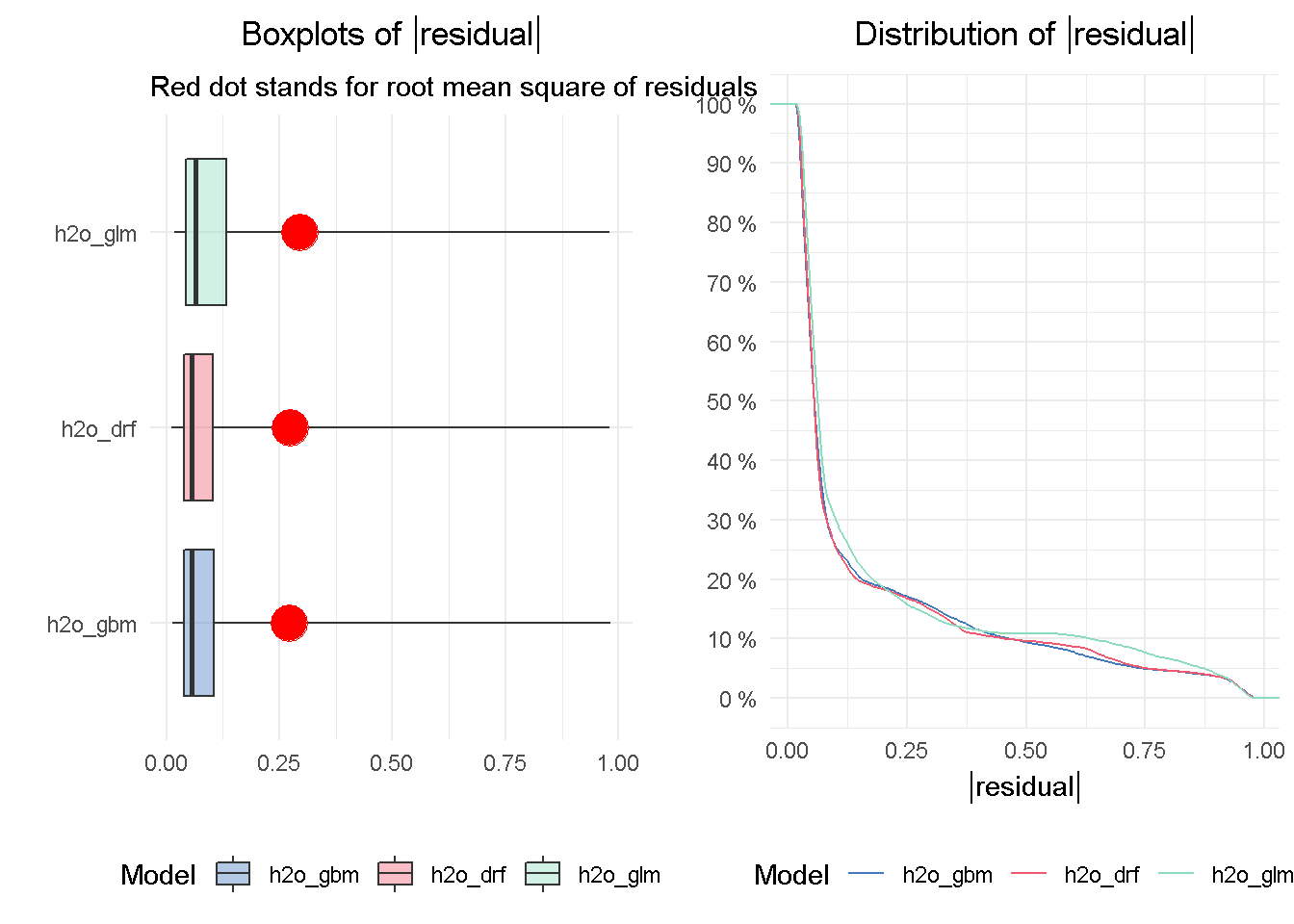The DRF and GBM models appear to perform on a par with one another, given the median absolute residuals. Looking at the residuals distribution on the right-hand side, you can see that the median residuals are the lowest for these two models, with the GLM seeing a higher number of tail residuals. This is also mirrored by the boxplots on the left-hand side, where the tree-based models both achieve the lowest median absolute residual value.

### 2 - How do the models compare with one another?

#### ROC and AUC

The Receiver Operating Characteristic (ROC) curve is a graphical method that allows to visualise a classification model performance against a random guess, which is represented by the striped line on the graph. The curve plots the true positive rate (TPR) on the y-axis against the false positive rate (FPR) on the x-axis.

``````eva_glm <- DALEX::model_performance(explainer_glm)
eva_dfr <- DALEX::model_performance(explainer_drf)
eva_gbm <- DALEX::model_performance(explainer_gbm)

plot(eva_glm, eva_dfr, eva_gbm, geom = "roc") +
ggtitle("ROC Curves - All Models",
"AUC_glm = 0.750  AUC_drf = 0.799  AUC_gbm = 0.798") +
theme_minimal() +
theme(plot.title = element_text(hjust = 0.5))
``````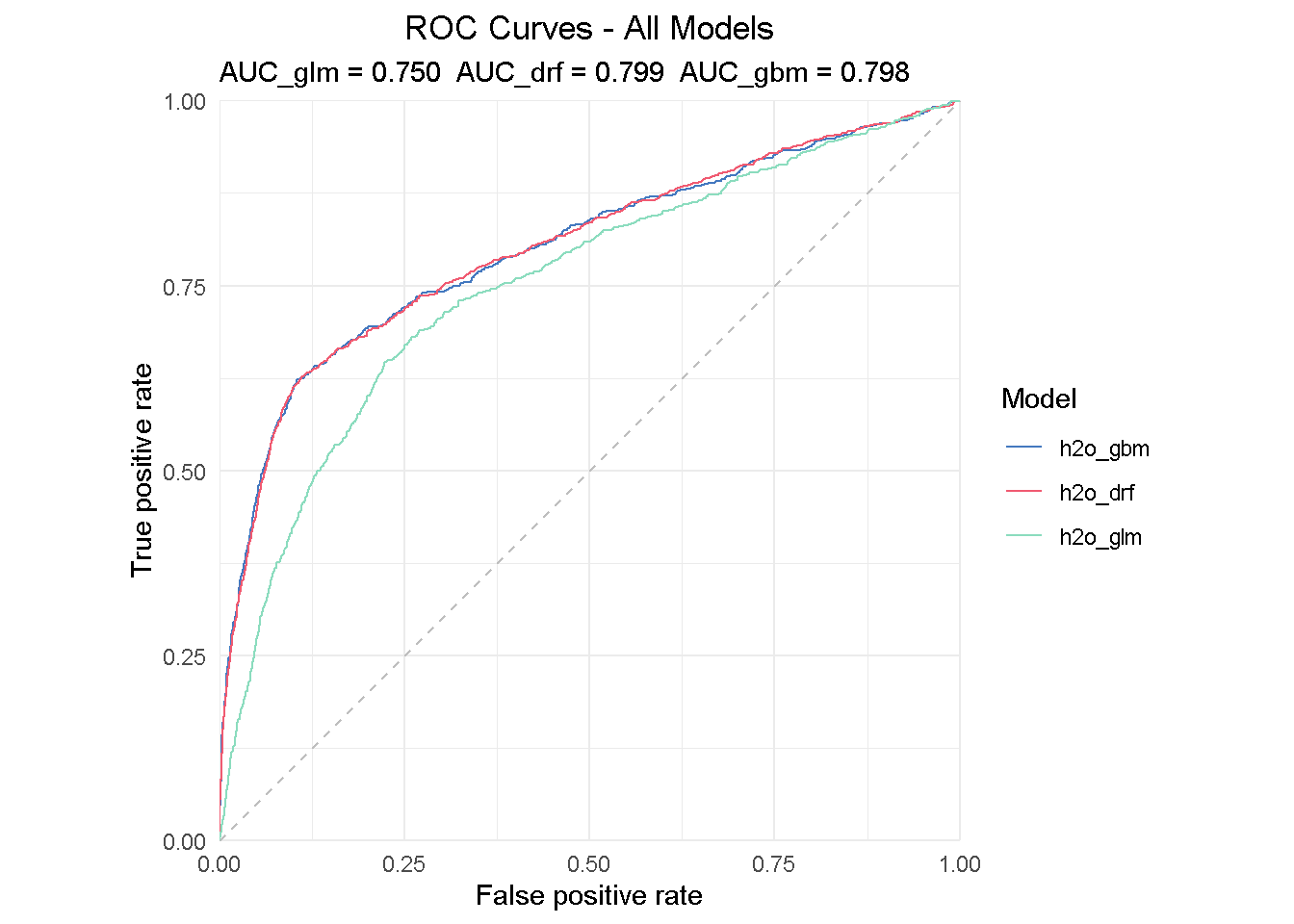The insight from a ROC curve is two-fold:

• Direct read: All models are performing much better than a random guess

• Comparing read: the AUC (Area Under the Curve) summarises the ROC curve and can be used to directly compare models performance - the perfect classifier would have AUC = 1.

All models performs much better that random guessing and achieves a AUC of .75-.80, with the DRF achieving the highest score of 0.799.

### 3 - Which variables are important in the models?

#### Variable importance plots

Each ML algorithm has its own way to assess the importance of each variable: linear models for instance refer to their coefficients, whereas tree-based models look at impurity, which makes it difficult to compare variable importance across models.

DALEX calculates variable importance measures via permutation, which is model agnostics, allowing for direct comparison between models of different structure. However, with variable importance scores based on permutations, we should remember that calculations slow down when the number of features increases.

Once again, I’m passing the “explainer” for each single model to the `feature_importance()` function and setting `n_sample` to 8000 to use practically all available observations. Although not exorbitant, the total execution time was nearly 30 minute but this is based on a relatively small dataset. But don’t forget that computation speed can be increased by reducing `n_sample`, which is especially important for larger datasets.

``````# measure execution time
tictoc::tic()

# compute permutation-based variable importance
vip_glm <- feature_importance(explainer_glm, n_sample = 8000,
loss_function = loss_root_mean_square)

vip_drf <- feature_importance(explainer_drf, n_sample = 8000,
loss_function = loss_root_mean_square)

vip_gbm <- feature_importance(explainer_gbm, n_sample = 8000,
loss_function = loss_root_mean_square)

# show total execution time
tictoc::toc()

## 1663.78 sec elapsed
``````

Now I only have to pass the vip objects to a plotting function: as suggested by the auto-generated x-axis label ( Drop-out loss), the main intuition behind how variable importance is calculated lies in how much the model fit would decrease if the contribution of a selected explanatory variable was removed. The larger the segment, the larger the loss when that variable is dropped from the model.

``````# plotting top 10 feature only for clarity of reading
plot(vip_glm, vip_drf, vip_gbm, max_vars = 10) +
ggtitle("Permutation variable importance",
"Average variable importance based on 8,000 permutations") +
theme_minimal() +
theme(plot.title = element_text(hjust = 0.5))
``````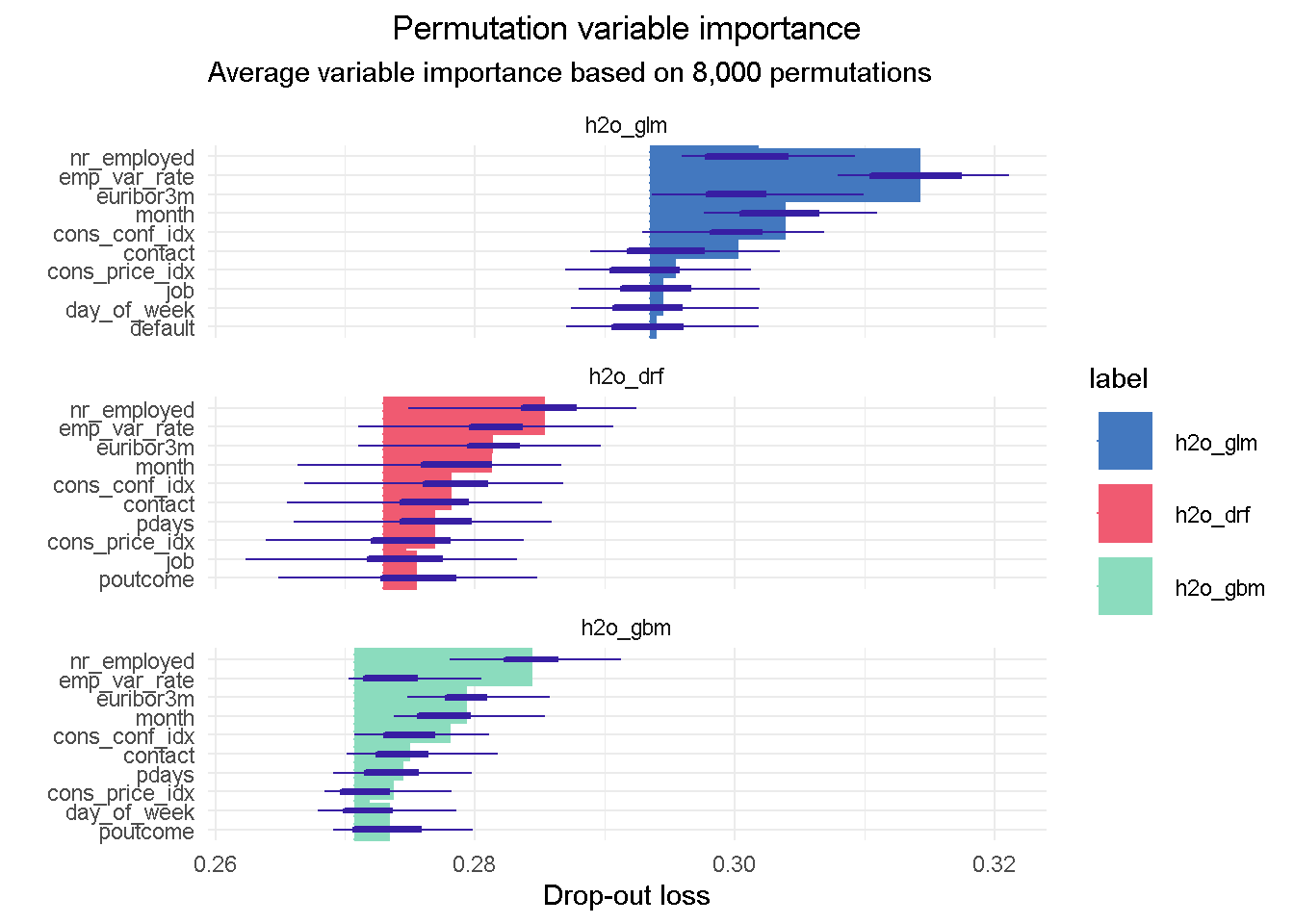I like this plot as it brings together a wealth of information!

First of all you can notice that, although with slightly different relative weights, the top 5 features are common to each models, with `nr_employed` ( employed in the economy) being the single most important predictor in all of them. This consistency is reassuring as it tells us that all models are picking up the same structure and interactions in the data, and gives us assurance that these features have strong predictive power.

You can also notice the distinct starting point for the x-axis left edge, which reflects the difference in the RMSE loss between the three models: in this case the elastic net model has the highest RMSE, suggesting the higher number of tail residuals seen earlier in the residual diagnostics is probably penalising the RMSE score.

### 4 - How does a single variable affect the average prediction?

#### Partial Dependence profiles

After we have identified the relative predictive power of each variable, we may want to investigate how their relationship with the predicted response differ across all three models. Partial Dependence (PD) plots, sometimes also referred to as PD profiles, offer a great way to inspect how each model is responding to a particular predictor.

We can start with having a look at the single most important feature, `nr_employed`:

``````# compute PDP for a given variable
pdp_glm  <- model_profile(explainer_glm, variable = "nr_employed", type = "partial")
pdp_drf  <- model_profile(explainer_drf, variable = "nr_employed", type = "partial")
pdp_gbm  <- model_profile(explainer_gbm, variable = "nr_employed", type = "partial")

plot(pdp_glm\$agr_profiles, pdp_drf\$agr_profiles, pdp_gbm\$agr_profiles) +
ggtitle("Contrastive Partial Dependence Profiles", "") +
theme_minimal() +
theme(plot.title = element_text(hjust = 0.5))
``````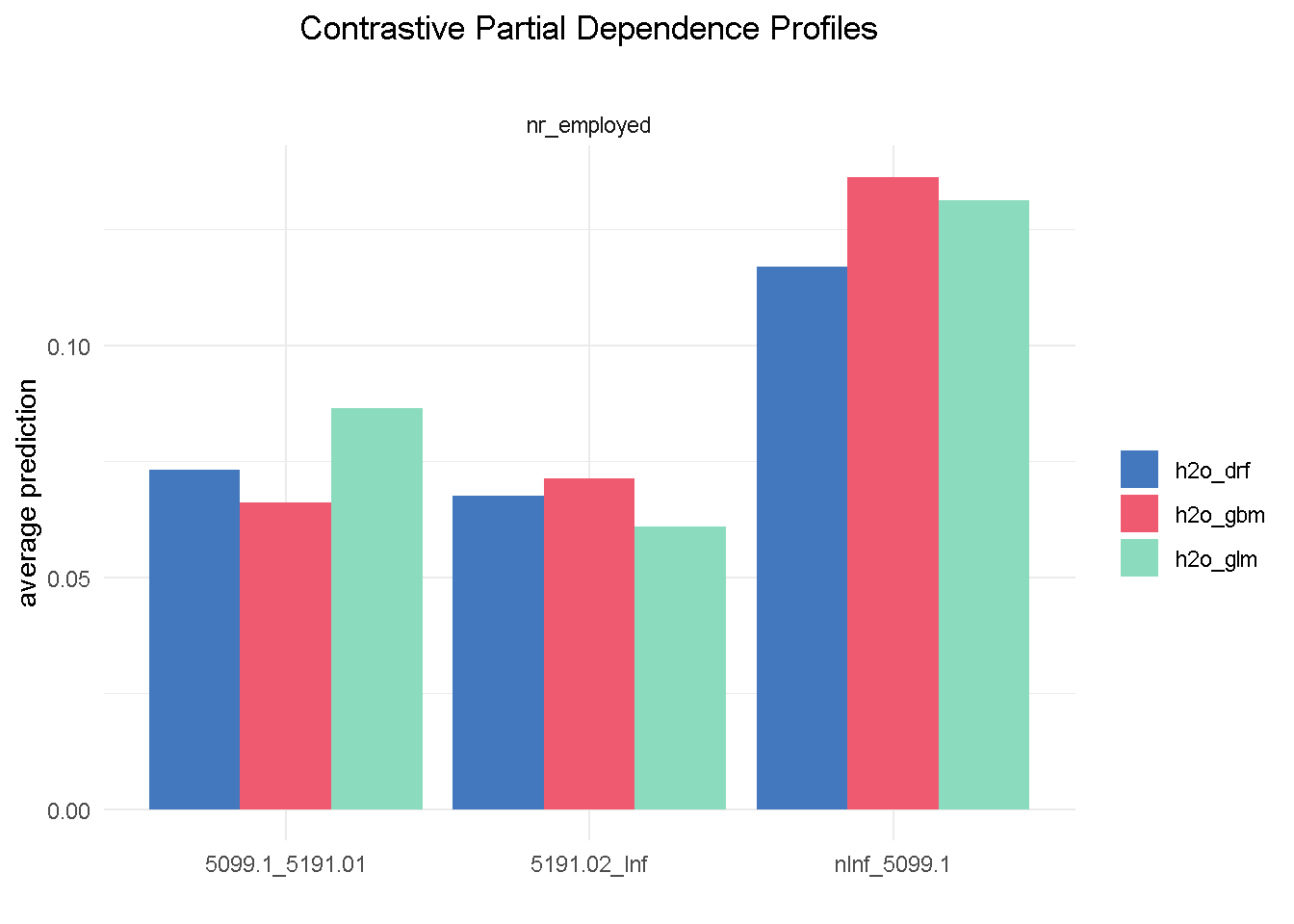Although with different average prediction weights, all three models found that bank customers are more likely to sigh up to a term deposit when the level of employed in the economy is up to 5.099m (`nInf_5099.1`). Both elastic net and random forest have found the exact same hierarchy of predictive power among the 3 different levels of `nr_employed` (less pronounced for the random forest) that we observed in the `correlationfunnel` analysis, with GBM being the one slightly out of kilter.

Let’s now take a look at `age`, a predictor that, if you recall from the EDA, was NOT expected to have an impact on the target variable:

``````# compute PDP for a given variable
pdp_glm  <- model_profile(explainer_glm, variable = "age", type = "partial")
pdp_drf  <- model_profile(explainer_drf, variable = "age", type = "partial")
pdp_gbm  <- model_profile(explainer_gbm, variable = "age", type = "partial")

plot(pdp_glm\$agr_profiles, pdp_drf\$agr_profiles, pdp_gbm\$agr_profiles) +
ggtitle("Contrastive Partial Dependence Profiles", "") +
theme_minimal() +
theme(plot.title = element_text(hjust = 0.5))
``````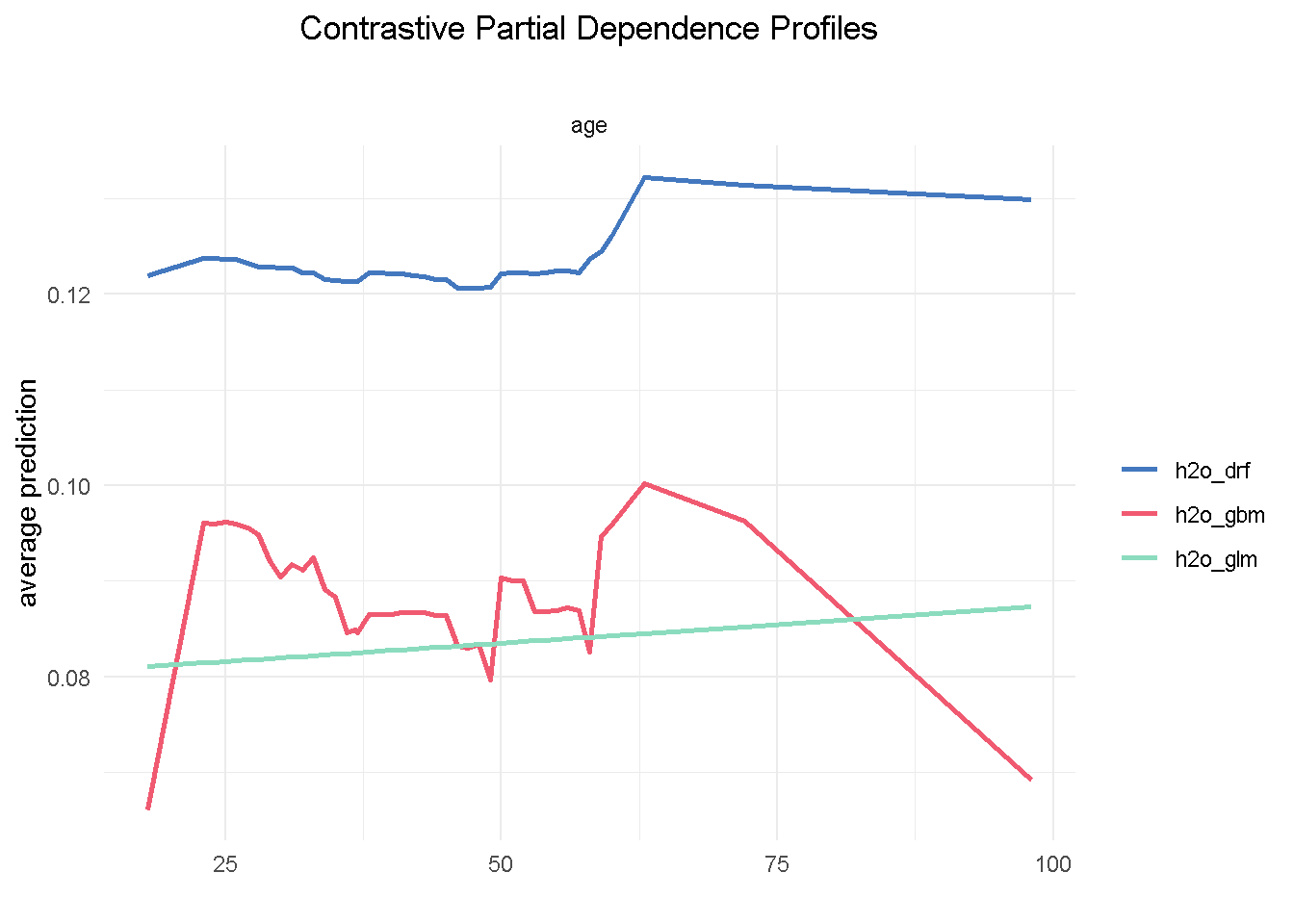One thing we notice is that the range of variation in the average prediction (x-axis) is relatively shallow across the age spectrum (y-axis), confirming the finding from the exploratory analysis that this variable would have a low predictive power. Also, both GBM and random forest are using `age` in a non-linear way, whereas the elastic net model is unable to capture this non-linear dynamic.

Partial Dependence plots could also work as a diagnostic tool: looking at `poutcome` (outcome of the `previous` marketing campaign) reveals that GBM and random forest correctly picked up on a higher probability of signing up when the outcome of a previous campaign was success (`scs`).

``````# compute PDP for a given variable
pdp_glm  <- model_profile(explainer_glm, variable = "poutcome", type = "partial")
pdp_drf  <- model_profile(explainer_drf, variable = "poutcome", type = "partial")
pdp_gbm  <- model_profile(explainer_gbm, variable = "poutcome", type = "partial")

plot(pdp_glm\$agr_profiles, pdp_drf\$agr_profiles, pdp_gbm\$agr_profiles) +
ggtitle("Contrastive Partial Dependence Profiles", "") +
theme_minimal() +
theme(plot.title = element_text(hjust = 0.5))
``````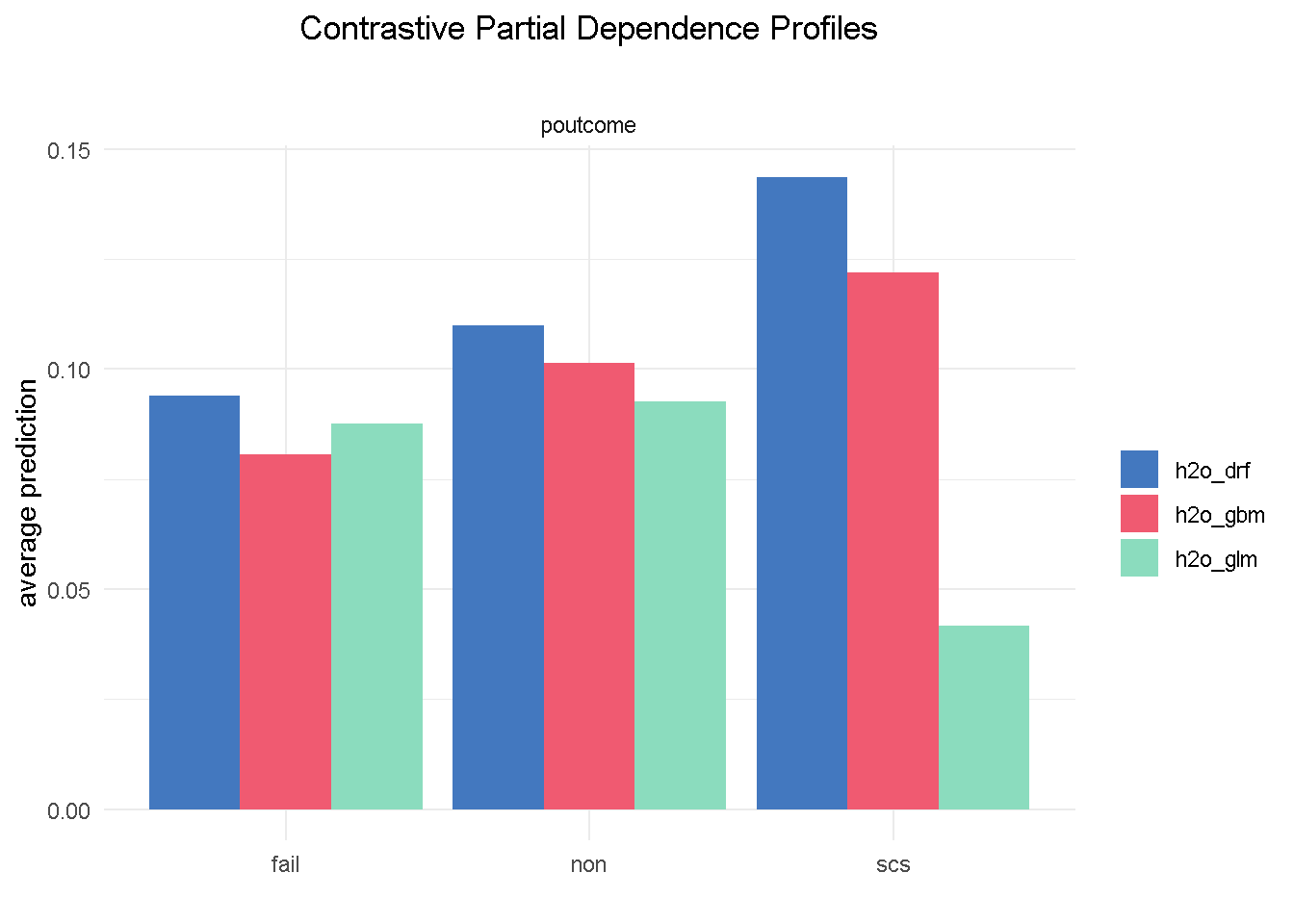However, the elastic net model fails to do the same, which could represents a serious flaw as success in a previous campaign had a very strong positive correlation with the target variable.

I’m going to finish with the `month` feature as it offers a great example of one of those cases where you may want to override the model’s outcome with industry knowledge and some common sense. Specifically, the GBM model seems to suggest that March, October and December are periods associated with much better odds of success.

``````# compute PDP for a given variable
pdp_glm  <- model_profile(explainer_glm, variable = "month", type = "partial")
pdp_drf  <- model_profile(explainer_drf, variable = "month", type = "partial")
pdp_gbm  <- model_profile(explainer_gbm, variable = "month", type = "partial")

plot(pdp_glm\$agr_profiles, pdp_drf\$agr_profiles, pdp_gbm\$agr_profiles) +
ggtitle("Contrastive Partial Dependence Profiles", "") +
theme_minimal() +
theme(plot.title = element_text(hjust = 0.5))
``````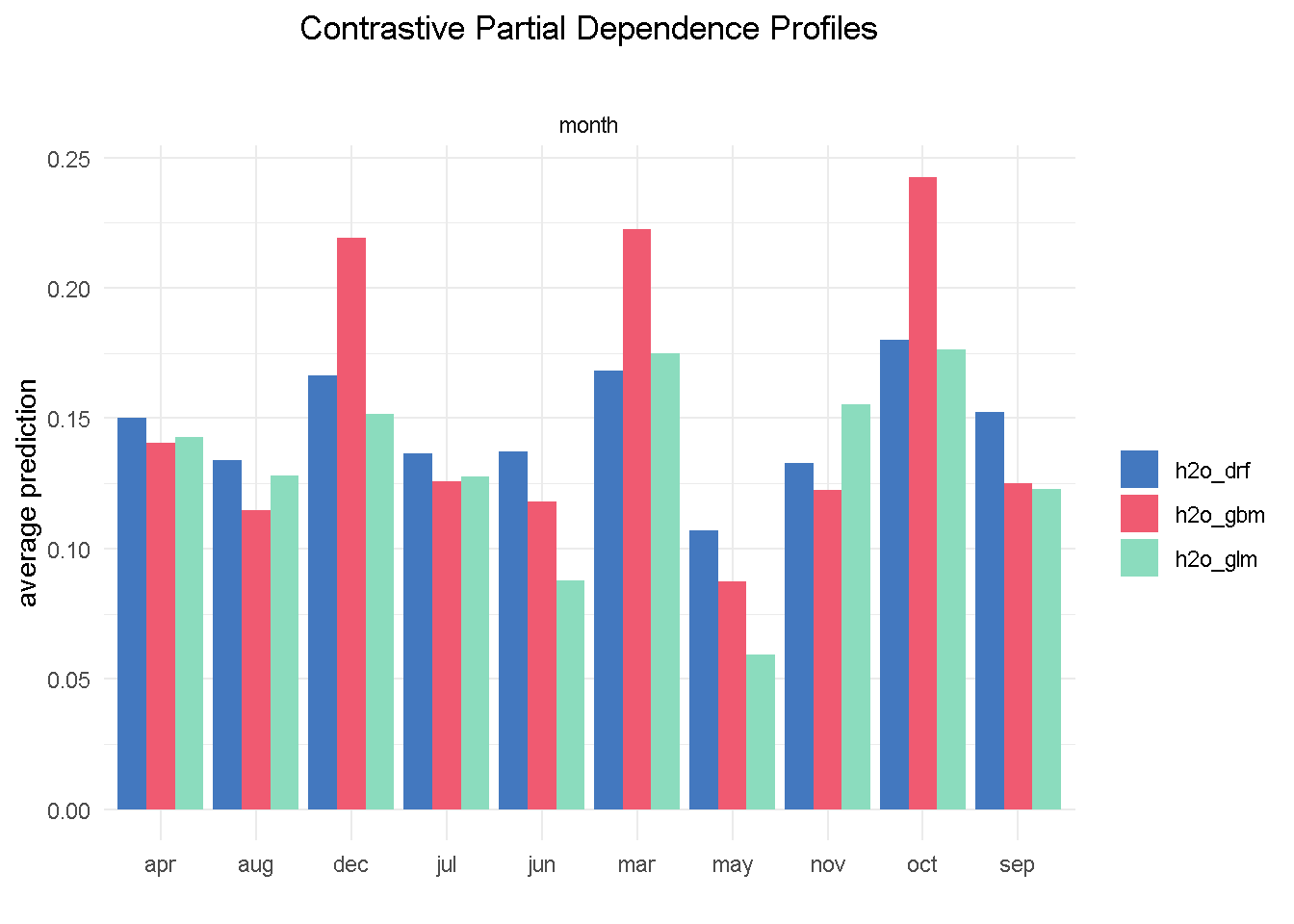Based on my previous analysis experience of similar financial products, I would not advise a banking organisation to ramp up their direct marketing activity around the weeks in the run to Christmas as this is a period of the year where the consumers’ focus shifts away from this type of purchases.

### Final model

All in all random forest is my final model of choice: it appears the more balanced of the three and does not display some of the “oddities” seen with variables like `month` and `poutcome`.

I can now further refine my model and reduce its complexity by combining findings from the Exploratory analysis, insight from models’ assessment and a number of industry-specific/common sense considerations.

In particular, my final model:

• Excludes a number of features (`age`, `housing`, `loan`, `campaign`, `cons_price_idx`) that have low predictive power

• Removes `previous`, which shows little difference between its 2 levels in the PD plot - it’s also moderately correlated with `pdays`, suggesting that they may capturing the same behaviour

• Also drops `emp_var_rate` because of its strong correlation with `nr_employed` and also because conceptually they are controlling for a very similar economic behaviour

.

``````# response variable remains unaltered
y <- "subscribed"

# predictors set: remove response variable and 7 predictors
x_final <- setdiff(names(train_tbl %>%
select(-c(age, housing, loan, campaign, previous,
cons_price_idx, emp_var_rate)) %>%
as.h2o()), y)
``````

For the final model, I’m using the same specification as to the original random forest

``````# random forest model
drf_final <-
h2o.grid(
algorithm       = "randomForest",
x               = x_final,
y               = y,
training_frame  = train_tbl %>% as.h2o(),
balance_classes = TRUE,
nfolds          = 10,
ntrees          = 1000,
grid_id         = "drf_grid_final",
hyper_params    = hyper_params_drf,
search_criteria = search_criteria_all,
seed            = 1975
)
``````

Once again, we sort the model by AUC score and retrieve the lead model

``````# Get the grid results, sorted by AUC
drf_grid_perf_final <-
h2o.getGrid(grid_id = "drf_grid_final",
sort_by = "AUC",
decreasing = TRUE)

# Fetch the top DRF model, chosen by validation AUC
drf_final <-
h2o.getModel(drf_grid_perf_final@model_ids[])
``````

Always remember to save your models!

``````# set path to get around model path being different from project path
path = "../03_models/"

# Save Final RF model
h2o.saveModel(drf_final, path)
``````

#### Final model evaluation

For brevity, I am visualising the variable importance plot with the `vip()` function from the namesake package, which returns the ranked contribution of each variable.

``````vip::vip(drf_final, num_features = 12) +
ggtitle("Variable Importance", "") +
theme_minimal() +
theme(plot.title = element_text(hjust = 0.5))
``````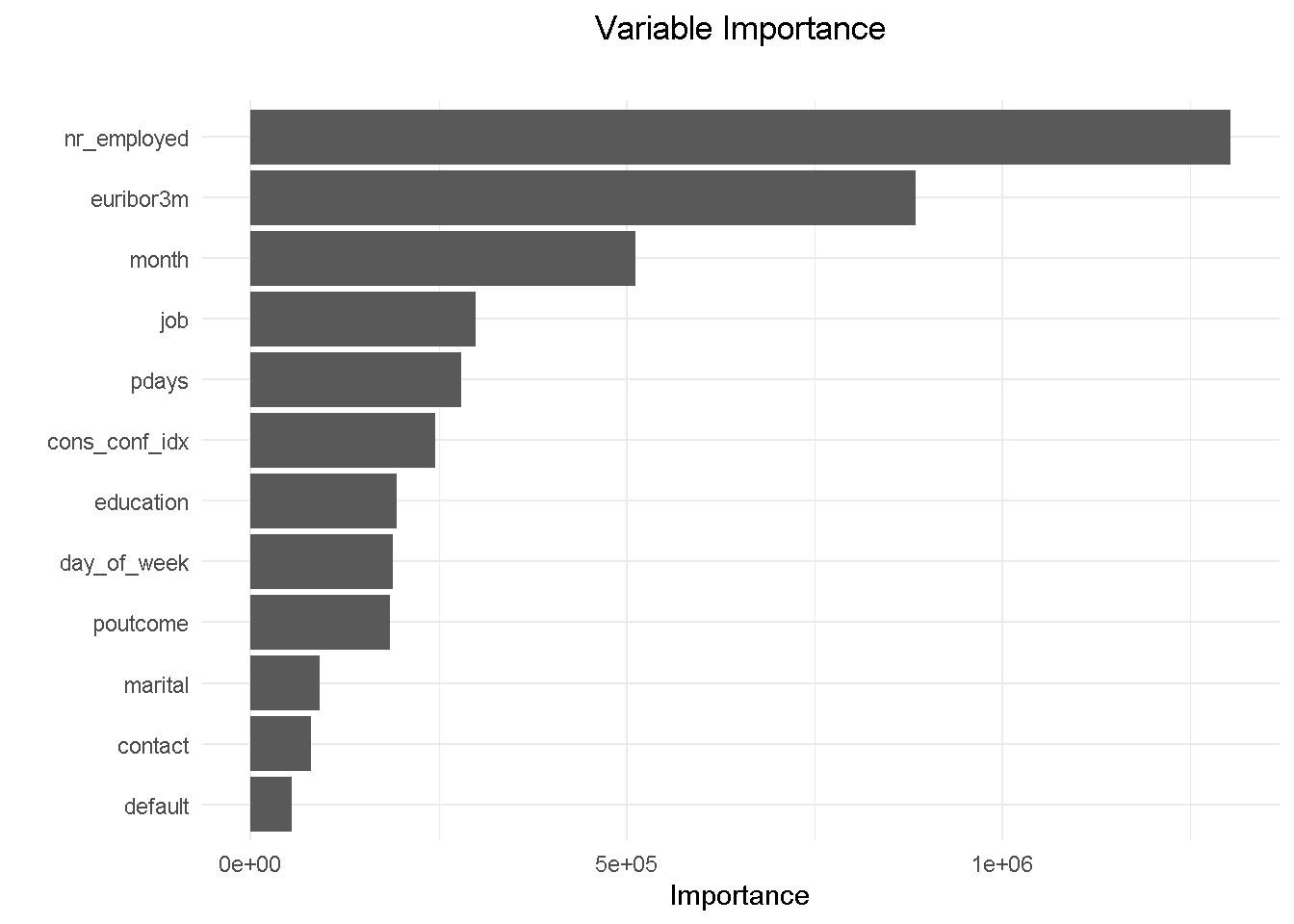Removing `emp_var_rate` has allowed `education` to come into the top 10 features. Understandably, the variables hierarchy and relative predictive power has adjusted and changed slightly but it’s reassuring to see that the other 9 variables were in the previous model’s top 10.

Lastly, I’m comparing the model’s performance with the original random forest model.

``````drf_final %>% h2o.performance(newdata = test_tbl %>% as.h2o()) %>% h2o.auc()

##  0.7926509
``````
``````drf_model %>% h2o.performance(newdata = test_tbl %>% as.h2o()) %>% h2o.auc()

##  0.7993973
``````

The AUC has only changed by a fraction of a percent, telling me that the model has maintained its predictive power.

### A important observation on Partial Dependence Plots

Being already familiar with odds ratios in the context of a logistic regression, I set out to understand whether the same intuition could be extended to black-box classification models. During my research one very interesting post on Cross Validated stood out for drawing a parallel between odds ratio from decision tree and random forest.

Basically, this tells us that Partial Dependence plots can be used in a similar way to odds ratios to define what characteristics of a customer profile influence his/her propensity to performing a certain type of behaviour.

For example, features like `job`, `month` and `contact` would provide context around who, when and how to target:

• Looking at `job` will tell us that a customer in an admin role is roughly 25% more likely to subscribe that a self employed.

• Getting in touch with a prospective customer in the `month` of October will more then double the chance of a positive outcome than in May.

• `contacting` your customer on their mobile increases the chances of subscription by nearly a quarter compared to a telephone call.

NOTE THAT Partial Dependence Plots for all final model’s predictors can be found in the Appendix

Armed with such insight, one can help shaping overall marketing and communication plans to focus on customers more likely to subscribe to a term deposit.

However, these are based on model-level explainers, which reflect an overall, aggregated view. If you’re interested to understand how a model yields a prediction for a single observation (i.e. what factors influence the likelihood to engage at single customer level), you can resort to the Local Interpretable Model-agnostic Explanations (LIME) method that exploits the concept of a “local model”. I will be exploring the LIME methodology in a future post.

### Summary of model estimation and assessment

For the analysis part of this project I opted for h2o as my modelling platform. h2o is not only very easy to use but also has a number of built-in functionalities that help speeding up data preparation: it takes care of class imbalance with no need for pre-modelling resampling, automatically “binarises“ character/factor variables, and implements cross-validation without the need for a separate `validation frame` to be “carved out” of the training set.

After setting up a random grid to search for best hyper-parameters, I’ve estimated a number of models ( a logistic regression, a random forest and a gradient boosting machines) and used the DALEX library to assess and compare their performance through an array of metrics. This library employs a model-agnostic approach that enables to compare traditional “glass-box” models and “black-box” models on the same scale.

My final model of choice is the random forest, which I further refined by combining findings from the exploratory analysis, insight gathered from the models’ evaluation and a number of industry-specific/common sense considerations. This ensured a reduced model complexity without compromising on predictive power.

## Closing thoughts

In the past decade machine learning has gained prominence across many analytics fields and has become a stable presence in the data scientist’s toolkit. Along with it, the field of Machine Learning Interpretability has gathered momentum and witnessed the flourishing of many libraries (like IML, PDP, VIP, and DALEX to name but the more popular) that help with feature explanation and general performance assessment.

I this project I’ve explored in particular the DALEX package, which focuses on Model-Agnostic Interpretability and provides a convenient way of comparing performance across multiple models with different structures.

One of its key advantages is the ability to compare contributions of traditional “glass-box” models as well as black-box models on the same scale. However, being permutation-based, one of its main drawbacks is that it does not scale well to large number of predictors and larger datasets.

Depending on the scale of your dataset and number of explanatory variables you want/need to include in your model, you should carefully consider whether to make DALEX your Machine Learning Interpretability library of choice.

### Code Repository

The full R code and all relevant files can be found on my GitHub profile @ Propensity Modelling

## Appendix

### Final Model PD Profiles

Update DALEX explainer inputs for the final model

``````# convert feature variables to a data frame
x_final <- test_tbl %>% select(-c(subscribed, age, housing, loan, campaign,
previous, cons_price_idx, emp_var_rate)) %>% as_tibble()

# change response variable to a numeric binary vector
y_final <- as.vector(as.numeric(as.character(test_tbl\$subscribed)))
``````

Create an explainer for the final model

``````# final random forest model explainer
explainer_final <- explain(
model            = drf_final,
type             = "classification",
data             = x_final,
y                = y_final,
predict_function = pred,
label            = "h2o_drf"
)
``````

Compute PD Plots for all final model’s features

``````# compute PDP for a given variable
model_profile(explainer_final, variable = "nr_employed", type = "partial") %>%
plot() +
theme_minimal() +
theme(plot.title    = element_text(hjust = 0.5),
plot.subtitle = element_text(hjust = 0.5))
``````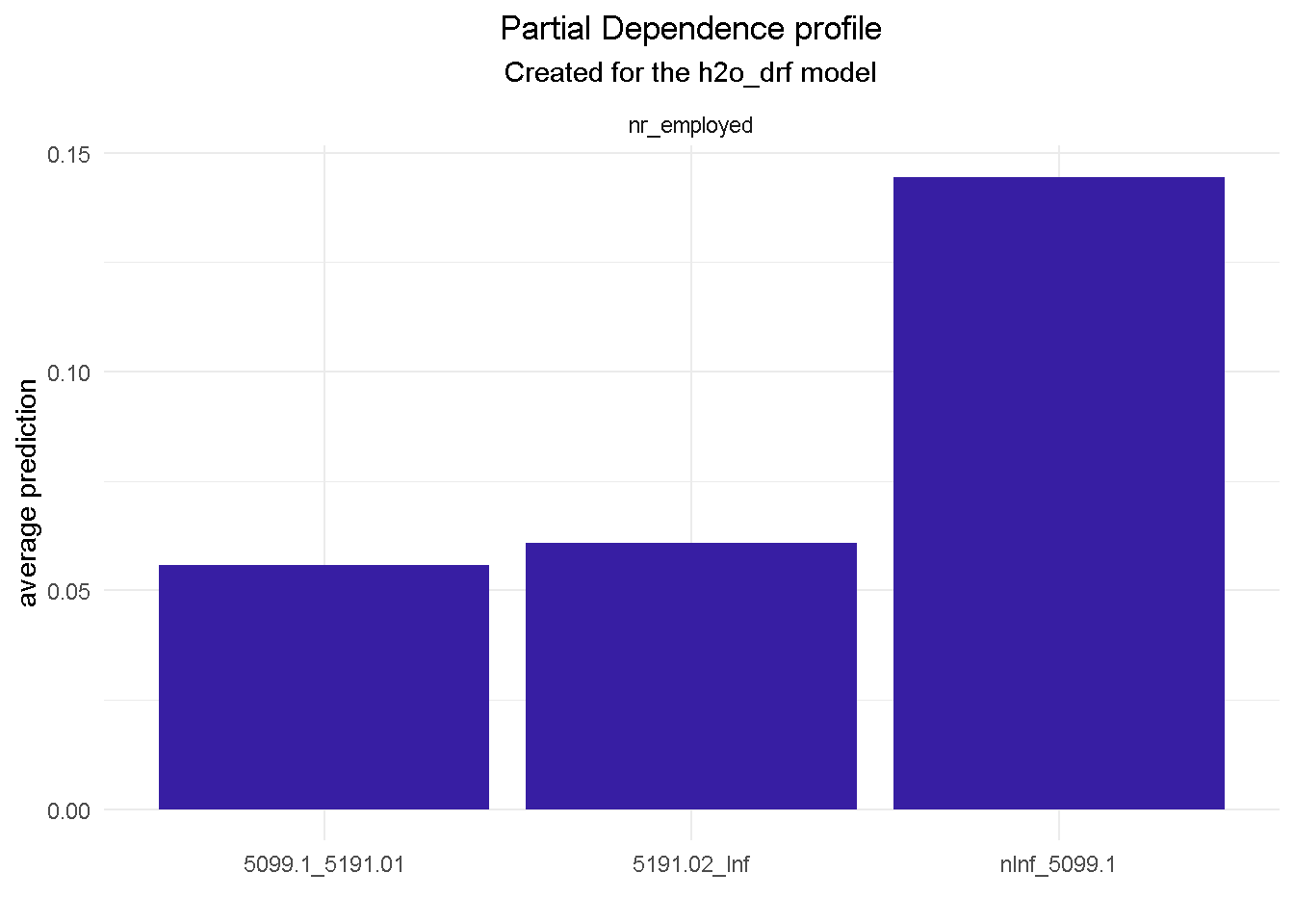``````model_profile(explainer_final, variable = "euribor3m", type = "partial") %>%
plot() +
theme_minimal() +
theme(plot.title    = element_text(hjust = 0.5),
plot.subtitle = element_text(hjust = 0.5))
``````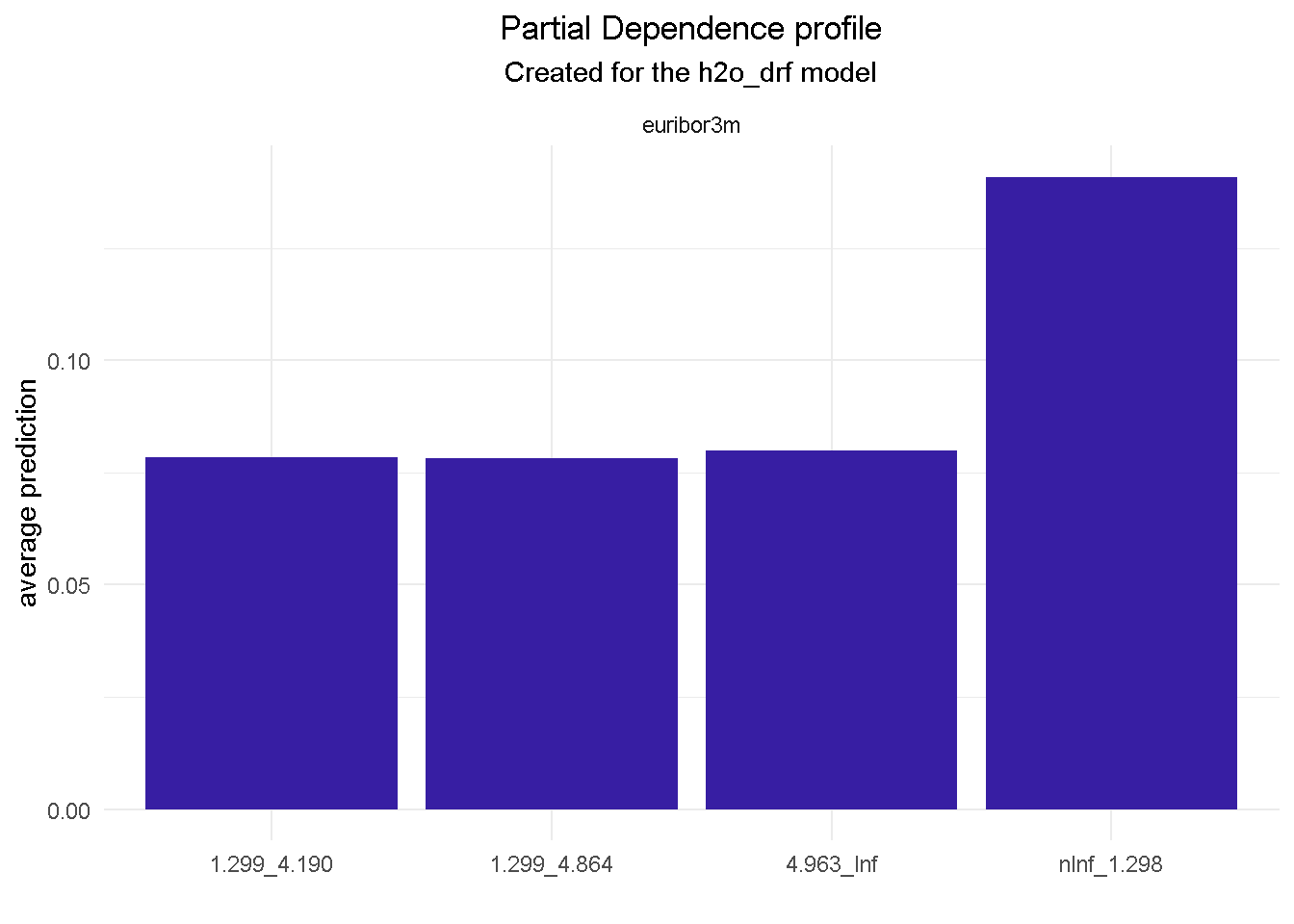``````model_profile(explainer_final, variable = "month", type = "partial") %>%
plot() +
theme_minimal() +
theme(plot.title    = element_text(hjust = 0.5),
plot.subtitle = element_text(hjust = 0.5))
``````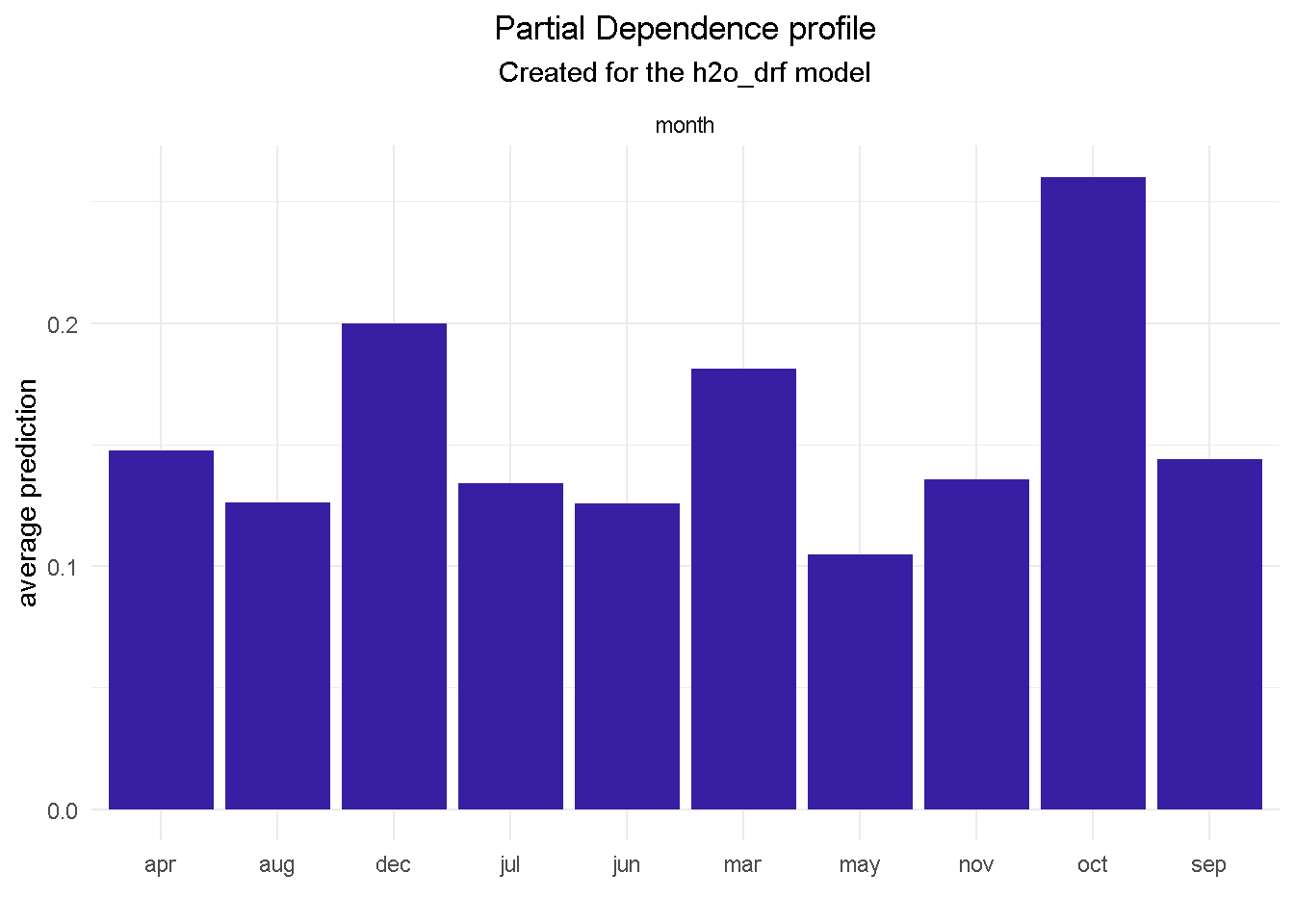``````model_profile(explainer_final, variable = "job", type = "partial") %>%
plot() +
theme_minimal() +
theme(plot.title    = element_text(hjust = 0.5),
plot.subtitle = element_text(hjust = 0.5))
``````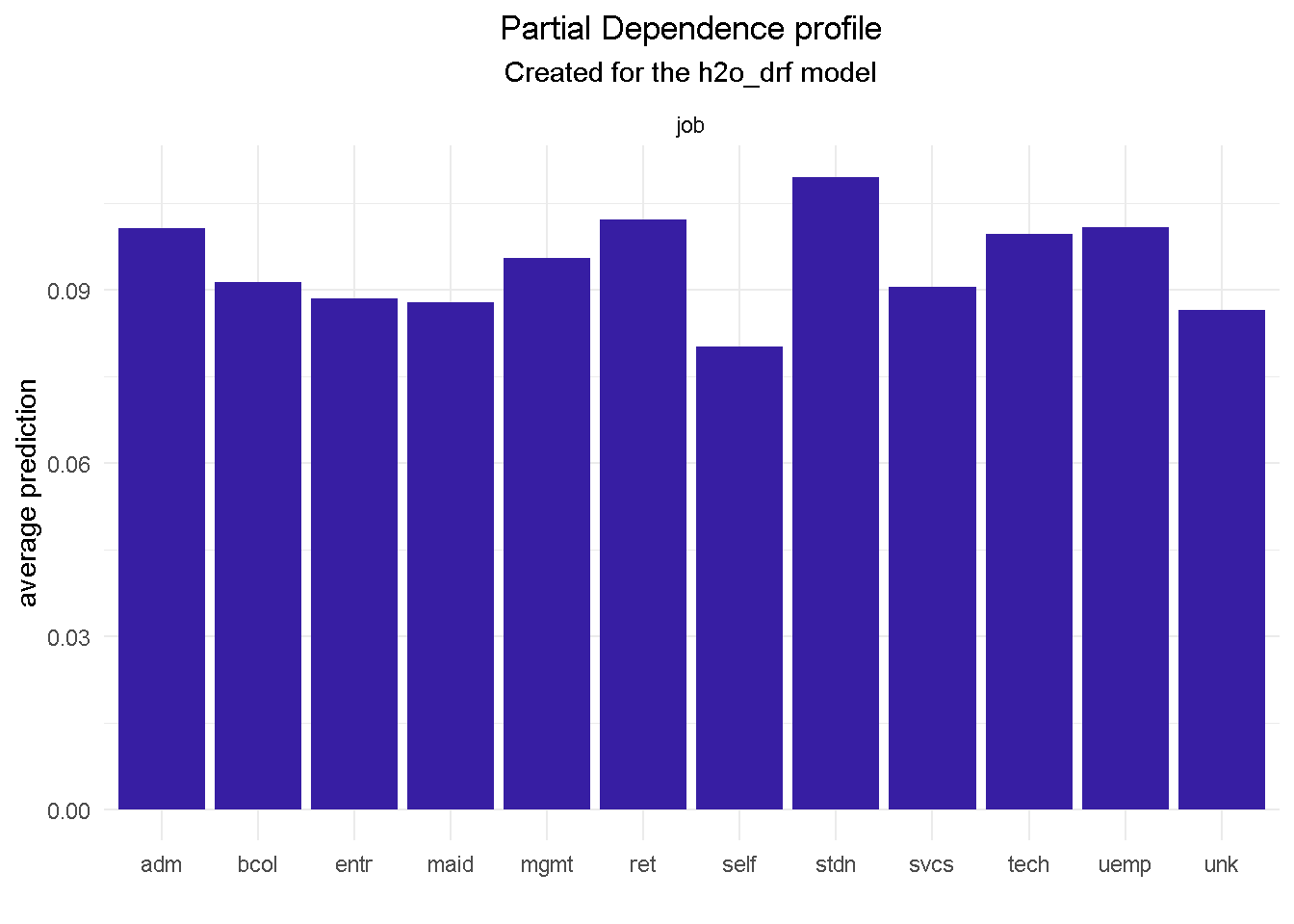``````model_profile(explainer_final, variable = "pdays", type = "partial") %>%
plot() +
theme_minimal() +
theme(plot.title    = element_text(hjust = 0.5),
plot.subtitle = element_text(hjust = 0.5))
``````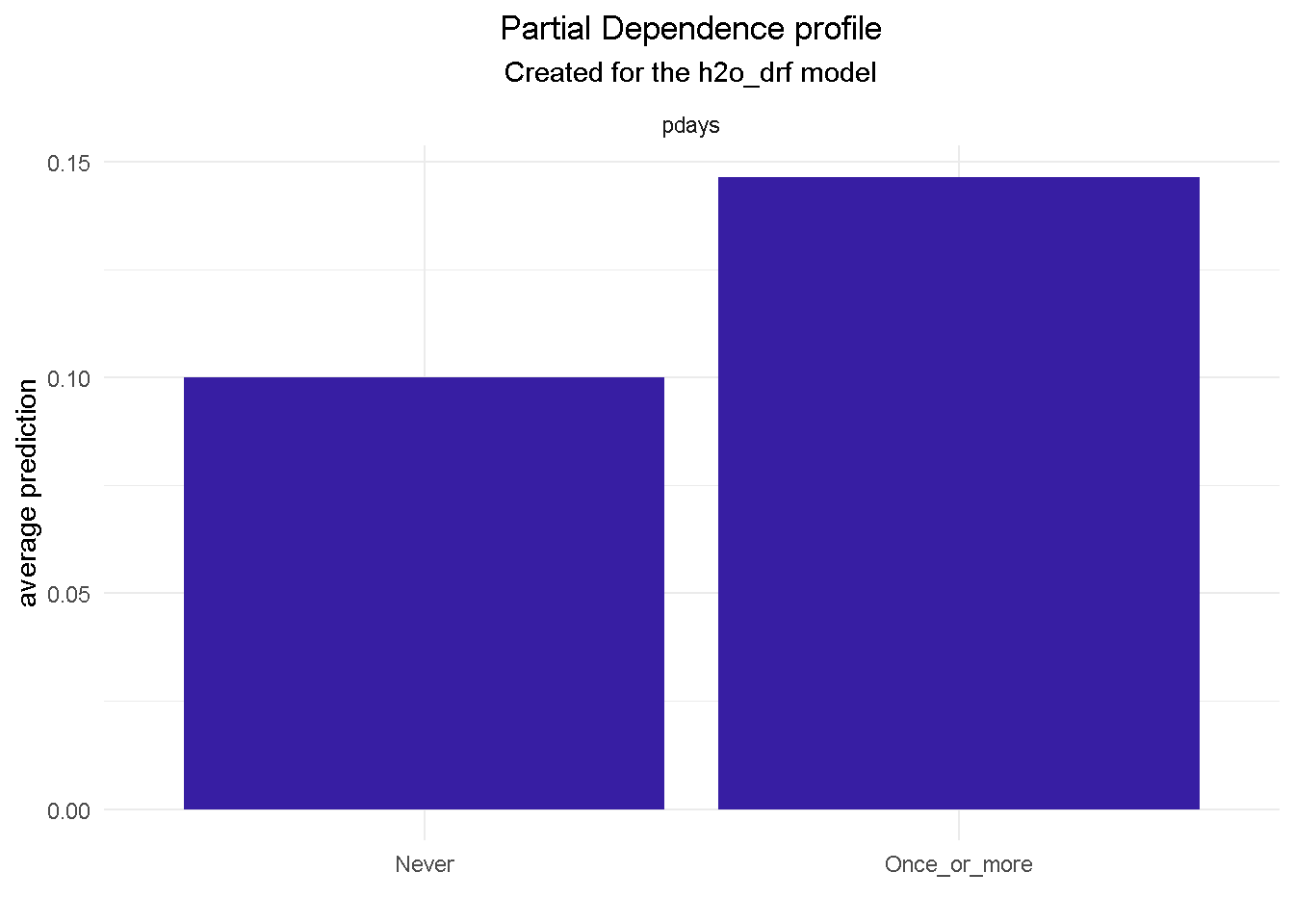``````model_profile(explainer_final, variable = "cons_conf_idx", type = "partial") %>%
plot() +
theme_minimal() +
theme(plot.title    = element_text(hjust = 0.5),
plot.subtitle = element_text(hjust = 0.5))
``````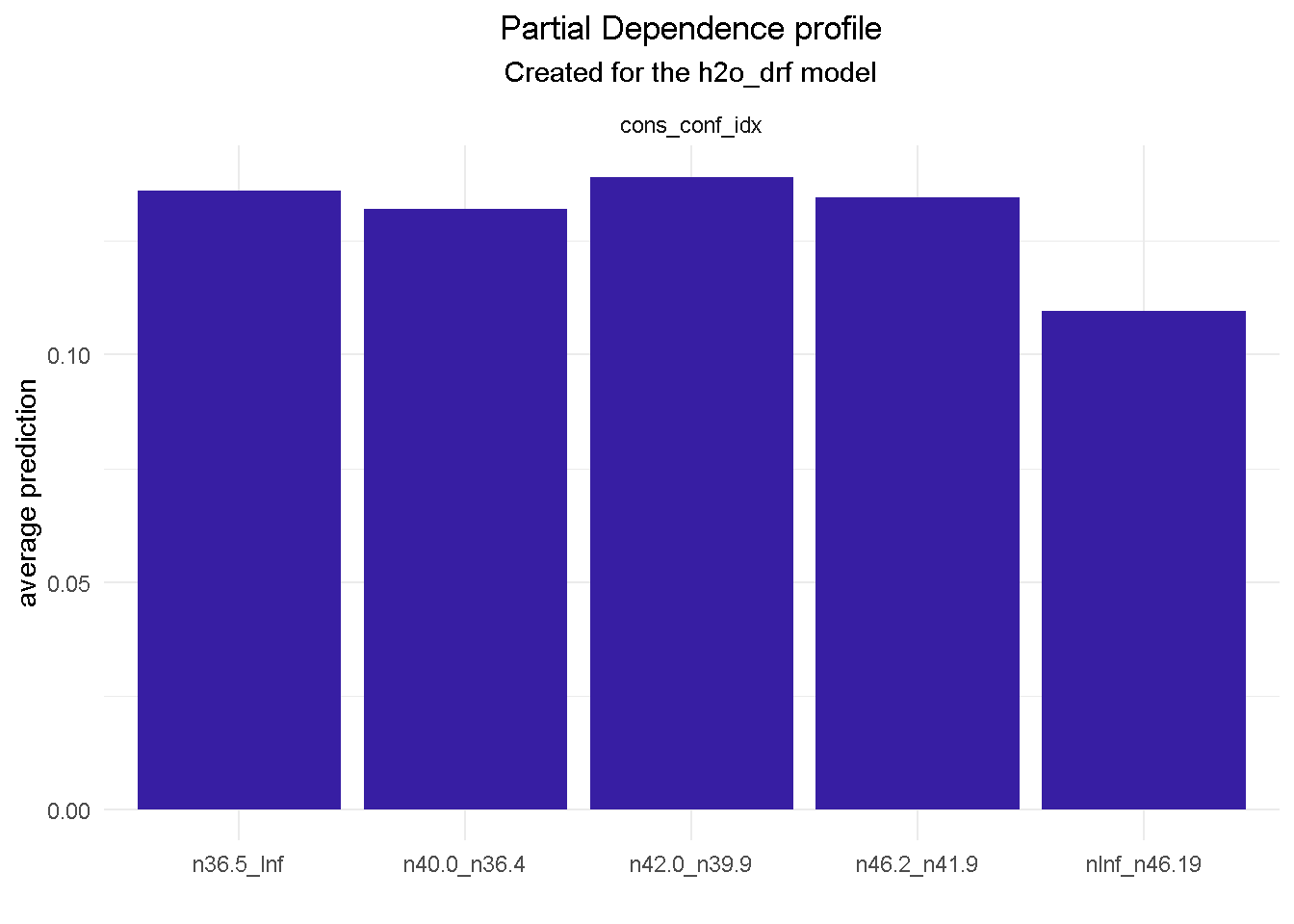``````model_profile(explainer_final, variable = "education", type = "partial") %>%
plot() +
theme_minimal() +
theme(plot.title    = element_text(hjust = 0.5),
plot.subtitle = element_text(hjust = 0.5))
``````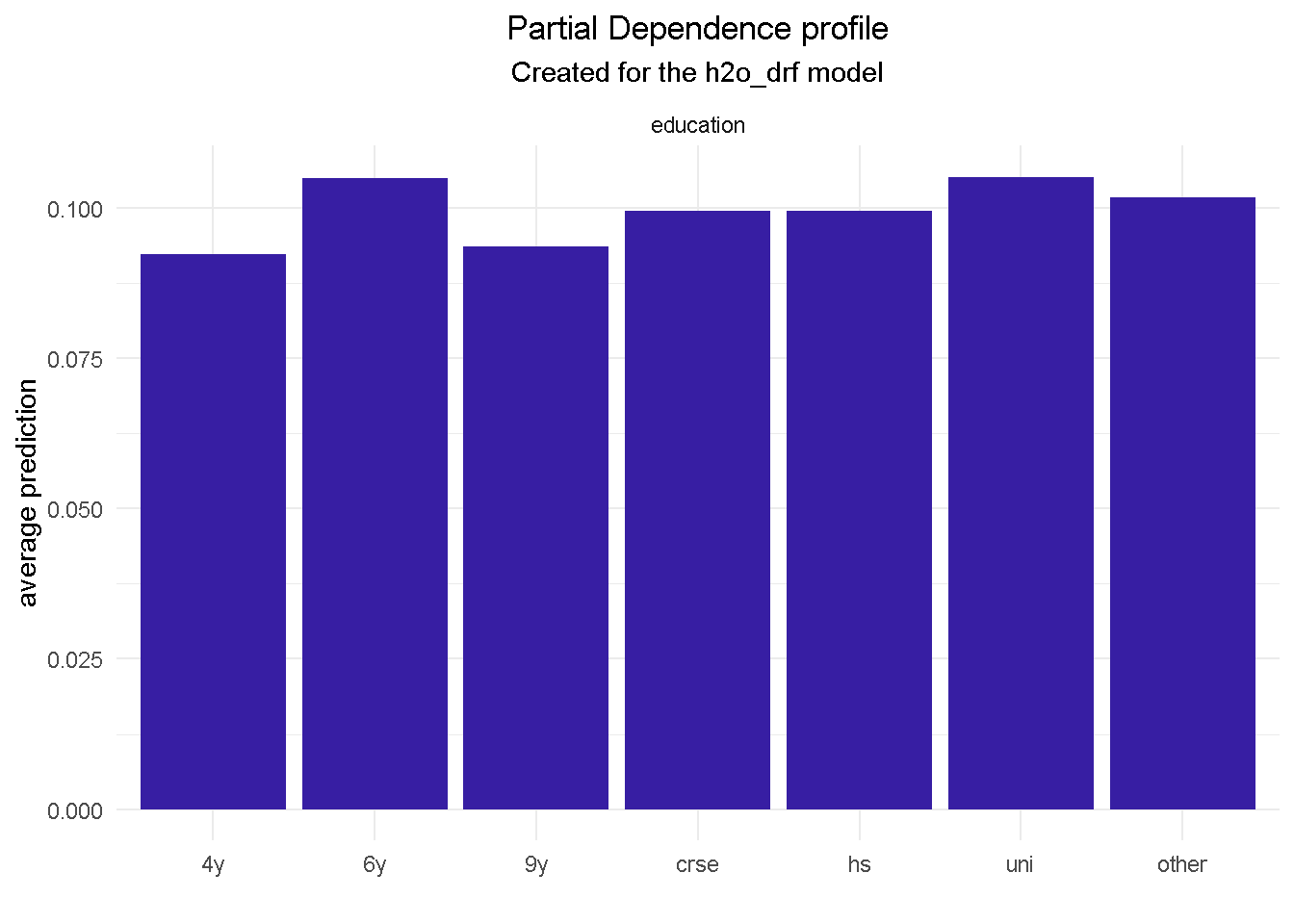``````model_profile(explainer_final, variable = "day_of_week", type = "partial") %>%
plot() +
theme_minimal() +
theme(plot.title    = element_text(hjust = 0.5),
plot.subtitle = element_text(hjust = 0.5))
``````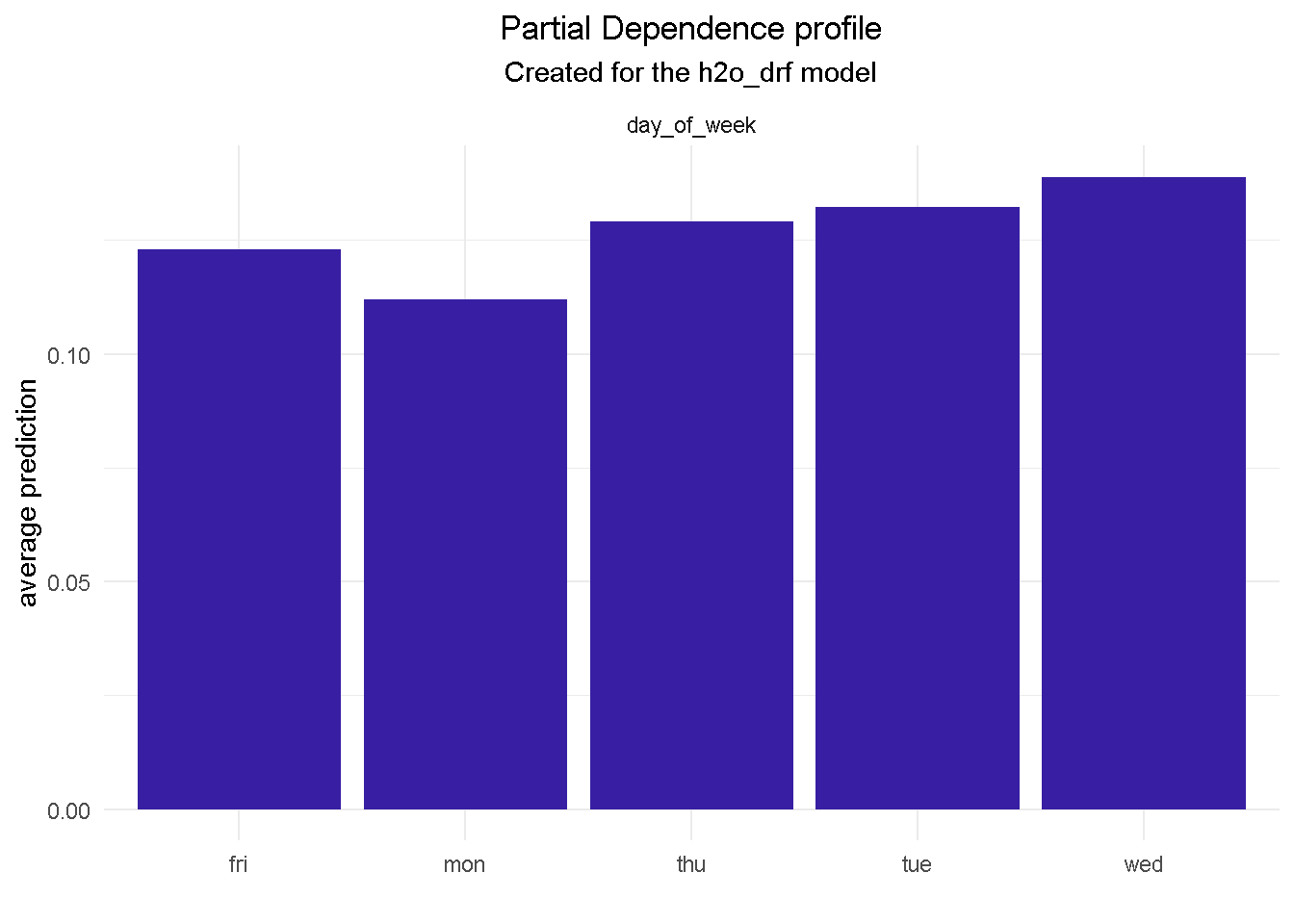``````model_profile(explainer_final, variable = "poutcome", type = "partial") %>%
plot() +
theme_minimal() +
theme(plot.title    = element_text(hjust = 0.5),
plot.subtitle = element_text(hjust = 0.5))
``````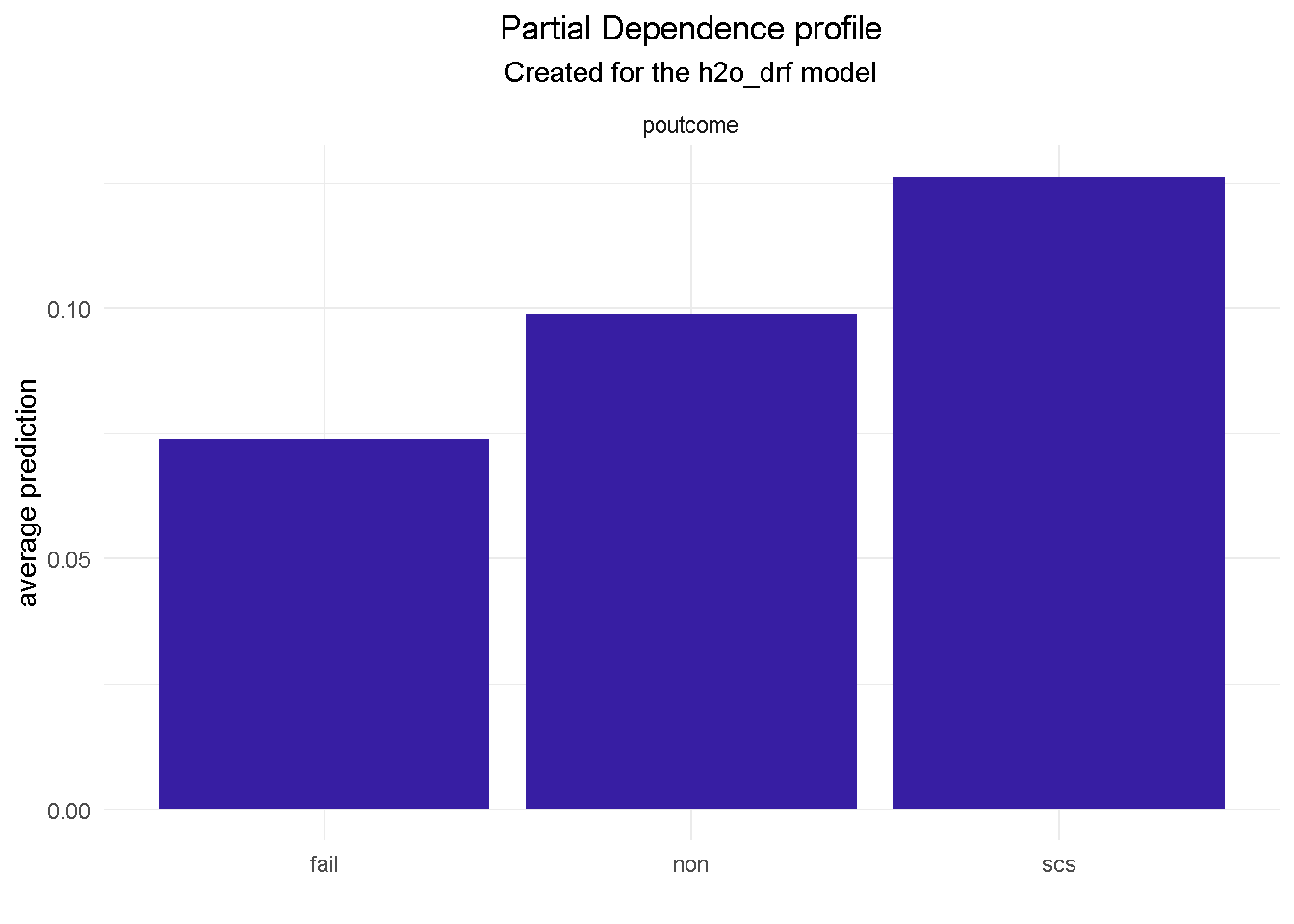``````model_profile(explainer_final, variable = "contact", type = "partial") %>%
plot() +
theme_minimal() +
theme(plot.title    = element_text(hjust = 0.5),
plot.subtitle = element_text(hjust = 0.5))
``````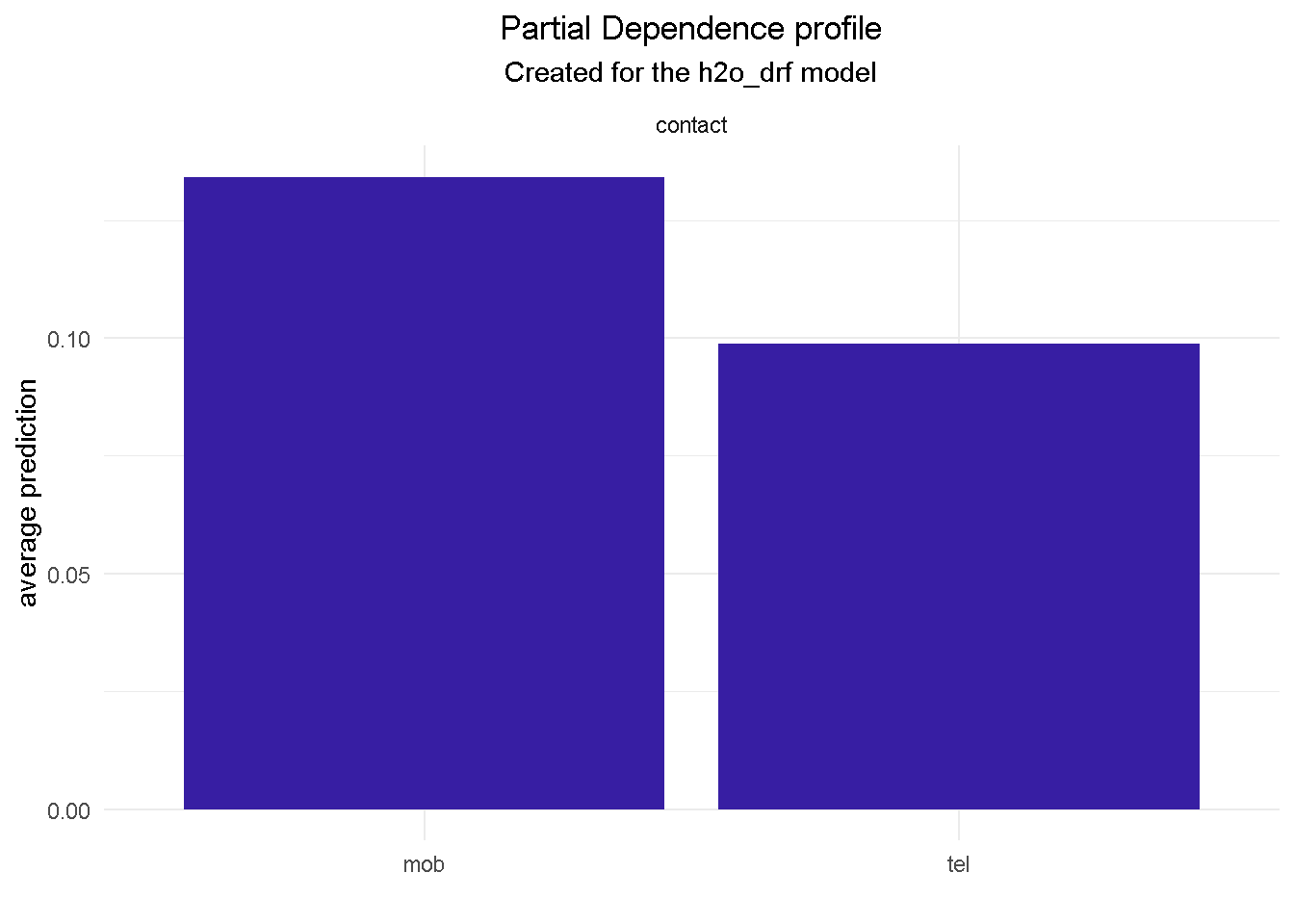``````model_profile(explainer_final, variable = "marital", type = "partial") %>%
plot() +
theme_minimal() +
theme(plot.title    = element_text(hjust = 0.5),
plot.subtitle = element_text(hjust = 0.5))
``````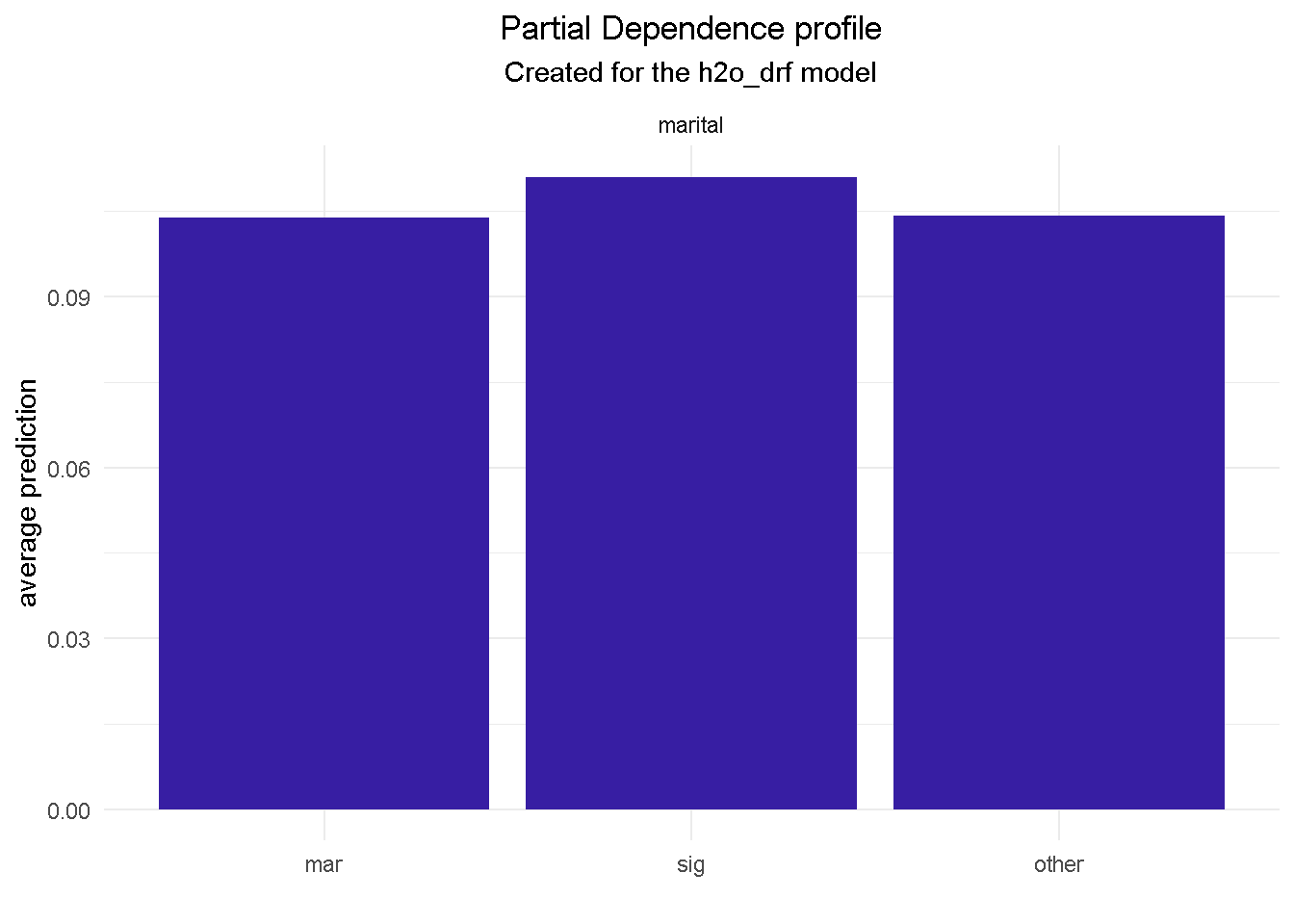``````model_profile(explainer_final, variable = "default", type = "partial") %>%
plot() +
theme_minimal() +
theme(plot.title    = element_text(hjust = 0.5),
plot.subtitle = element_text(hjust = 0.5))
``````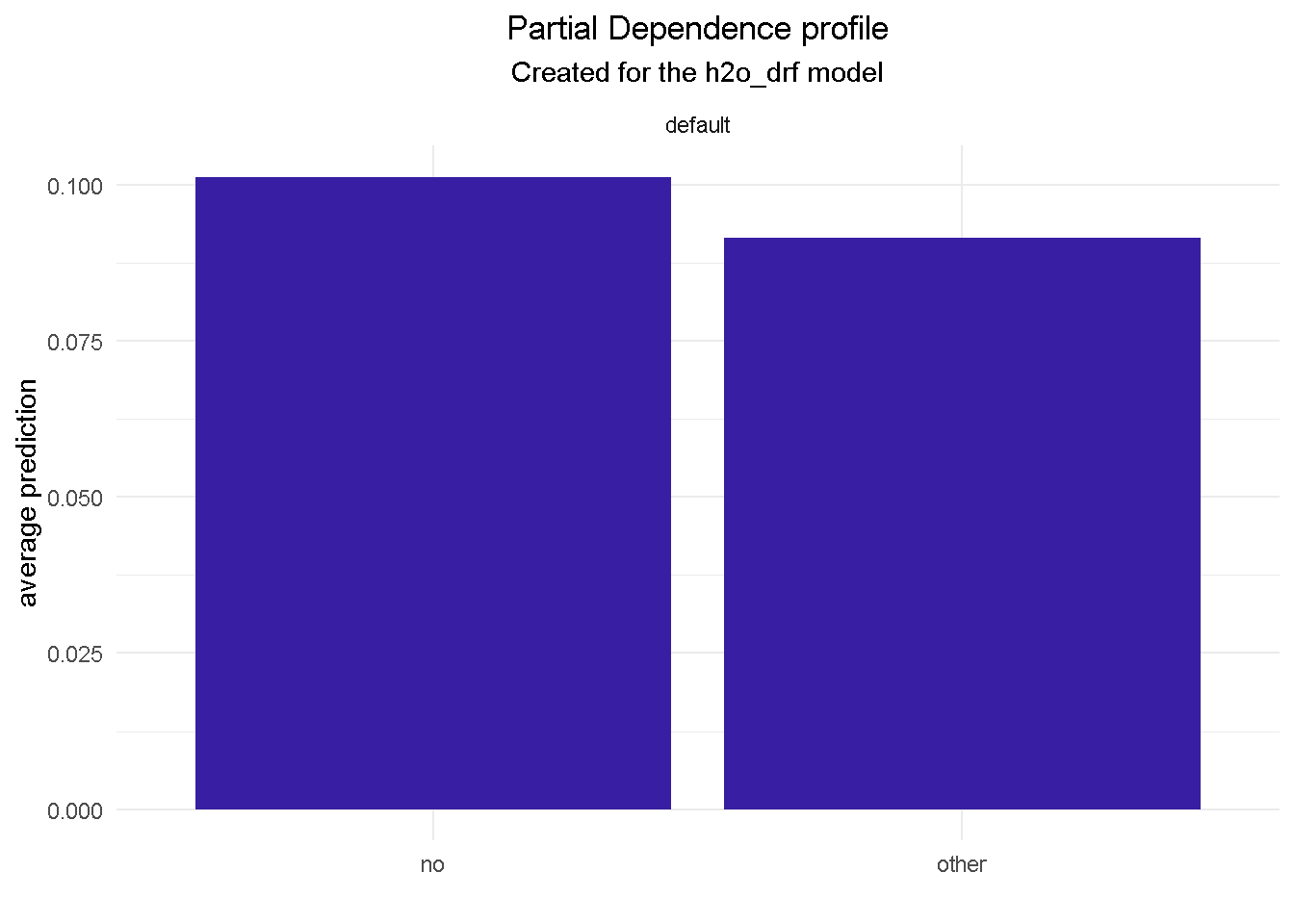One final thing: don’t forget to shut-down the h2o instance when you’re done!

``````h2o.shutdown(prompt = FALSE)
``````